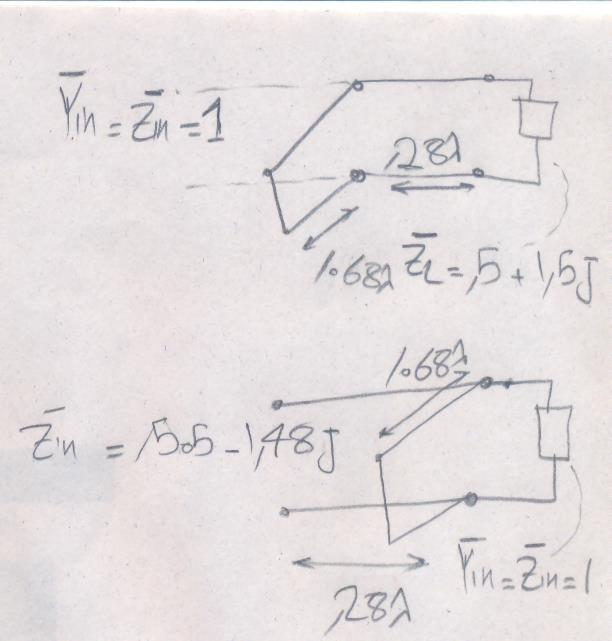Single Stub matching

1. Single Stub matching

Hello
As you see this problem with single stub matchingI matched zl=.5+1.5i to a normalized line (Z0=50)(I did it with smith chart and analytically) but as you see in the second picture as I calculate Zin it doesn't get me the same result (.5+1.5j).
what is my wrong?•

2. Re: Single Stub matching

Are you going in the same direction around the smith chart?•

3. Re: Single Stub matching

Hello
Yes I did•

4. Re: Single Stub matching

The impedance of the shorted stub reduces to z_in = j*tan(2*pi*1.68) = j2.13.

The input impedance of the load as viewed from the stub is z_in = ((0.5+j1.5) + j(50Ω)*tan(2*pi*0.28))/((50Ω) + j(0.5+j1.5)*tan(2*pi*0.28)) = 0.21 - j4.49.

The two in parallel don't give 1... am I missing something?5. Re: Single Stub matching

I am a little confused. Why do you keep handwriting "Yin = Zin"? Yin is of course 1/Zin

and in computing the impedance where the stub joins the main line, the ADMITTANCES add, not the impedances. You want normalized Yin total to equal 1 at that point.

1 members found this post helpful.•

6. Re: Single Stub matching

Hello dear biff44
"Yin=Zin" it means that if we find normalized Zin=1 then Yin=1/Zin=1.